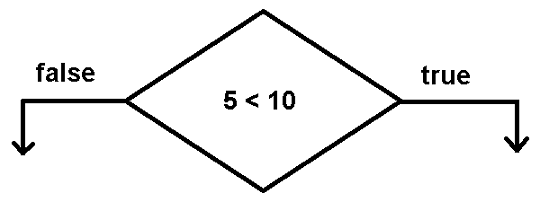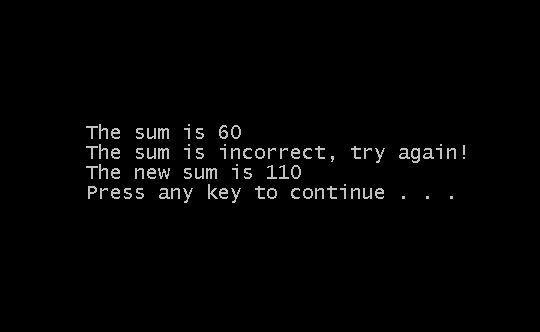## C Programming Tutorial – Flow Control Structures – I

In a C program, the statements are executed sequentially – one at a time. But, not all C programs are executed in the same way. In some programs, we want to execute a part of the code and ignore others parts.

The flow of the program must change when we meet certain criteria or not meet the criteria. This is can be achieved using flow control structure in C language. A flow control structure in a C program can change the direction of flow of the program depending on the conditions. In this way, a certain section of code is ignored within a C program.

A list of flow control structure is given below.

1. If statements
2. If-else statements
3. Switch-case statements
4. Conditional statements

Relational Operators are used for setting conditions within flow control structures.

The list of relational operator with brief description is given below.

Operator Description
==Is equal to
!=Is not equal to
<=Less than or equal to
>=Greater than or equal to
<Less than
>Greater than

If Statements

The ‘if’ block will execute a one or more C statements when a condition is true.
The syntax for if block is as follows

``````if (condition)

{

statement 1;

}``````

There will not be any execution if the condition is false. The relational operator with the operands will evaluate to true or false.

For example

``````if ( 5 < 10)

{

printf (“ The statement is true”);

}

``````

In the above example, 5 < 10 is true by default and evaluate to true. Hence, the output will be “The statement is true”.

## Types of if blocks

In this section we will discuss types of if block that you can use without getting errors in a C program.
Single if block with no curly brackets

``````if ( condition)
statement 1;
``````

Single if block with curly brackets

``````if ( condition)
{

statement 1;

}
``````

Single if – else block without curly brackets

``````if( condition)

Statement 1;

else

statement 2;``````

Single if – else block with curly brackets

``````if (condition)
{

statement 1;

statement 2;

}
else
{

statement 3;

statement 4;

}
``````

Single if – else if – else block with curly brackets

``````if ( condition)
{

statement 1;

statement 2;

}
else if ( condition 2)
{

statement 3;

statement 4;

}
else
{

statement 5;

}
``````

In the above block, there are two conditions that the program need to meet but it starts from the condition 1 and if the condition 1 is not met, checks for condition 2 and if that is not met either then execute the statement 5.

It is a good practice to use the curly bracket to avoid confusion.

The brackets show the boundaries for each block and brings clarity and also very helpful in managing codes when the program becomes very large.

Nested If Blocks

The nested block is blocked within another block and they are executed first whenever the block is executed. The following is an example of a nested block.

``````if ( condition 1)
{

statement 1;

if( condition 2)
{

statement 2;

statement 3;

}

}
``````

## Nested Blocks

In the example above, statement 2 and statement 3 are executed first when condition 2 is true. The nested block will not be executed if condition 1 is false in the parent block. The types of the nested block are given below.

Nested if block with curly brackets

``````if ( condition 1)
{

if( condition 2)
{

statement 1;

statement 2;

}

}
``````

Nested if block with no curly brackets

``````if ( condition 1)
{

if ( condition 2)

statement 1;

statement 2;

}
``````

Nested if – else block with curly brackets

``````if (condition 1)
{

if (condition 2)
{

statement 1;

statement 2;

}

else

{

statement 3;

statement 4;

}

}
``````

Nested if-else without curly brackets

``````if ( condition 1)
{

if( condition 2)

statement 1;

statement 2;

else

statement 3;

statement 4;

}
``````

Second Level nested ‘if’ block with curly brackets

``````if( condition 1)
{

if (condition)
{

statement 1;

if (condition 3)
{

statement 2;

statement 3;

}

}
else
{

statement 4;

}

}
``````

## Flowchart – Symbol

In a C program flowchart, the flow control such as if block is denoted by a diamond shape.Flow Control – If block diagram

## Examples – if – else and nested if blocks

In this section, an example of simple if block, if-else block and nested if block is given to demonstrate the usage of flow control structure in a C programs.

The example is written using Dev-C++ 4.9.9.2 compiler installed on a Windows 7 64-bit PC.

``````#include <stdio.h>

int main()
{

int number1, number2, number3, sum;

number1 = 20;

number2 = 40;

number3 = 50;

sum = 0;

sum = number1 + number2;

/* The ‘if’ block */

if(number3 < 60) { printf(“The sum is %d\n”, sum); }

/* The ‘if-else’ block */

if(sum == 70)
{

printf(“The sum is correct\n”);
}
else
{

printf(“The sum is incorrect, try again!\n”);

}

/* nested if block */

if ( sum > 50)
{

if( number3 > 40)
{

sum = sum + number3;

printf(“The new sum is %d\n”,sum);

}

}

system(“pause”);

return 0;

}
``````

## Output-Example if, if-else and nested if blockOutput-Flow-Control-If-else

## Bibliography

Balagurusamy, E. 2000. Programming in ANSI C. Tata McGraw-Hill Education,.

Brian W. Kernighan, Dennis M. Ritchie. 1988. C Programming Language, 2nd Edition. Prentice Hall.

Kanetkar, Yashavant. 20 November 2002. Let us C. Bpb Publications.